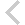FICO Xpress Optimization Examples Repository
 FICO Optimization Community FICO Xpress Optimization HomeSudoku (CP and MIP models)

Description
Playing Sudoku: fill in the 9x9 grid so that every row, every column and every 3x3 box contains the numbers 1-9.
• The models sudoku.mos and sudoku2_ka.mos solve a given Sudoku grid with Mixed Integer Programming and Constraint Programming respectively.
• The model versions sudoku*_graph.mos add repeated display of the Sudoku board to show the progress of the solving.

Source Files
By clicking on a file name, a preview is opened at the bottom of this page.

Data Files

sudoku.mos

(!****************************************************************
Mosel example problems
======================

file sudoku.mos

Sudoku puzzle - data read from file.

(c) 2008 Fair Isaac Corporation
author: S. Heipcke, Jul 2020
*****************************************************************!)

model "sudoku (MIP)"
uses "mmxprs", "mmsystem"

parameters
DATAFILE = "sudokug290705.dat"
end-parameters

forward procedure print_solution

declarations
XS = {'A','B','C','D','E','F','G','H','I'}
YS = 1..9
VALS = 1..9
VALUE: dynamic array(XS,YS) of integer
v: array(XS,YS) of mpvar
b: array(XS,YS,VALS) of mpvar
end-declarations

initializations from "Data/"+DATAFILE
VALUE
end-initializations

! Fix variables to the given values
forall(x in XS, y in YS | exists(VALUE(x,y))) v(x,y) = VALUE(x,y)

! Associate binaries with cell variables
forall(x in XS, y in YS) do
forall(i in VALS) b(x,y,i) is_binary
v(x,y) = sum(i in VALS) i*b(x,y,i)
sum(i in VALS) b(x,y,i) = 1
end-do

starttime:=gettime

! All-different values in rows
forall(y in YS, i in VALS) sum(x in XS) b(x,y,i) = 1

! All-different values in columns
forall(x in XS, i in VALS) sum(y in YS) b(x,y,i) = 1

! All-different values in 3x3 squares
forall(k in 0..2, i in VALS) do
sum(x in {'A','B','C'}, y in {1+3*k,2+3*k,3+3*k}) b(x,y,i) = 1
sum(x in {'D','E','F'}, y in {1+3*k,2+3*k,3+3*k}) b(x,y,i) = 1
sum(x in {'G','H','I'}, y in {1+3*k,2+3*k,3+3*k}) b(x,y,i) = 1
end-do

! Solve the problem
minimize(0)
if getprobstat=XPRS_OPT then
print_solution
end-if

writeln("Total time: ", gettime-starttime)

!****************************************************************
! Solution printing
procedure print_solution
writeln("Solution:")
write("   "); forall(x in XS) write(x," "); writeln
forall(y in YS) do
write(y, ": ")
forall(x in XS) write(v(x,y).sol," ")
writeln
end-do
returned:=true
end-procedure

end-model

`